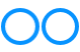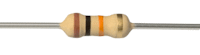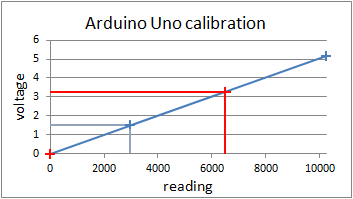# John Errington's Experiments with an Arduino

## Voltage measurement with the Arduino board: Calibration

### Why do we need to calibrate our voltmeter?

In the preceding chapters we have assumed the values of resistors are exactly correct. This of course is rarely true.
Common resistors are sold in "preferred values" chosen to cover the normal range of requirements. For example the E12 range (of 10% tolerance) has 12 values in each decade, as follows:

10R, 12R, 15R, 18R, 22R, 27R, 33R, 39R, 47R, 56R, 68R, 82R ; then their multiples 100R, 120R etc.

So a "47k" E12 resistor will have a tolerance of 10% or +- 4.7k

For best accuracy we can use higher spec (more expensive) resistors. The tolerance is shown by the color of the rightmost band as shown below.

E12: 10% tolerance (silver); E24: 5% tolerance (gold); E48: 2% tolerance(red); E96: 1% tolerance (brown)Even if we make our divider chain from E96 resistors, a chain of two resistors will have a possible error of 2 * 1% = 2%; to achieve the 0.25% accuracy the Arduino is capable of we need to make adjustments to compensate for these errors.

### How to calibrate the voltmeter

This sketch takes measurements from an analog input (here A0) and prints the results on the serial monitor. Note we take ten measurements and add them up. This reduces the effect of noise and gives us a more precise value.

Here I've used the "DEFAULT" (5V) reference for measurements and connected the input to the "3.3V" reference for calibration - but you could of course use the 4.096 or 2.500V external reference for more accurate results.

```/* uno: calibrate ADC inputs */ const int repeats = 10; // number of times to repeat the measurement long int sum = 0; // a running total of the measurements for that channel ```

```void setup() { Serial.begin(9600); analogReference(DEFAULT); // NB use "EXTERNAL" only if using an external reference }```

```// take measurements and print result void loop() { readvoltage(); Serial.print("sum of ten readings is : "); Serial.print(sum); Serial.println("; "); delay (2000); }```

```void readvoltage() { sum = 0; for (int j = 0; j < repeats; j++) {  sum += analogRead(0);  delay(7); // choose a short delay that does not add to 20msec.  } }```

#### The output will look something like this:

```sum of ten readings is : 6473; sum of ten readings is : 6475; sum of ten readings is : 6474;```

To get voltage measurements we need to change the scale to suit our particular arrangement of components. We start by connecting the voltmeter input to the 0V connection, and note our reading. If all is working correctly it should be near zero.

Then we connect the voltmeter input to a known voltage "Vcal" - and record the result "Rcal".
These readings are used to work out the scale factor required to convert the reading into a voltage.

### Example calibration and calculation of scaling factorUsing the DEFAULT reference (measured as 5.16V) my Uno gave readings of 0 for a 0V input, and Rcal = 6474 (as shown above) for an input of Vcal = 3275mV (the "3.3V" reference)

So for any reading R the voltage is V (mV) = R * 3275 / 6474.

To convert any reading at that input to a voltage in millivolts we multiply by our scale factor of 3275 / 6474 = 0.5059

I connected a battery at the input and the reading was 2950.
So the voltage would be V = 2950 * 3.275 / 6474 = 1492 mV.
My meter showed it was 1495 mV

You can see the calibration points marked in red on this graph, and the reading for the battery in blue.

if we amend the sketch like this

`const float scalefactor = 0.5059; // my scalefactor: yours will be different!`

``` float voltage = sum * scalefactor; // convert to voltage in millivolts Serial.print("voltage in mV is : "); Serial.print(voltage,0); ```

the result (shown below) is a reading that is correct to 0.25%

```voltage in mV is : 3275; voltage in mV is : 3275; ```